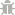Light Mode
Rate us!# Binary To HEX

Enter your Binary numbers with space:## Binary To Hexadecimal Converter – Complications Free!

Binary numbers today not only make the designing of computer and related technologies simple but also they are easy to understand by a machine. The most straightforward explanation of binary number system can be a machine representing numbers in the form of 0 and 1 instead of the digits 0-9.

The names in the binary are added to left as the digits get larger and for computers, it gets simplified when they are performing tasks. Also, binary numbers can be programmed on and off for a switch or a transistor which you can make a part of a calculator or any storage device.

If you aren’t aware, pictures and audio can also be turned into 0 and 1. First, they have to be turned into tiny elements which can be pixels and sample for sound; later every single pixel is assigned a unique number.

This is the very reason why this number system is most commonly used in computer engineering and networking. Whereas, Hex or call it Hexadecimal number system has the system of base 16; also, it is interesting in many ways as our decimal system uses only ten digits to represent numbers, hexadecimal uses sixteen. The six figures after ten are letters of the English alphabets, (A, B, C, D, E, and F). This number is used in two different places:

1. Information TechnologiesIn information technologies or math’s, each hex number represent four binary digits, and if there aren’t four digits, then extra zeros are added on the left to meet the condition. If you haven’t seen, in the URL (Uniform Resource Links) character codes are found with percentage prefixes; such numbers are also hexadecimal. In XML and Html, we use the Hexadecimal numbers for representing colors where each color is assigned a six-digit hex value. The IPV6 addresses can also be noted as eight groups of hex digits, where every group is divided or separated by a colon.
2. In MathematicsWhen it comes to solving problems and equations and to understand the purpose of hexadecimal characters Math is the subject for you. It is really difficult sometimes to turn binary numbers to hexadecimal characters when they have a large grouping of numbers. It takes a lot of time as the steps include the gathering of figures in four digits, if the long binary number can’t be separated in the group of fours then you will need to add extra zeros to complete the number. Later you will need to consult a chart of hex numbers if you haven’t added them to your memory.

You can avoid all of these steps by using our binary to hex online converter. This is where you can add long binary numbers for conversion to hex. Hexadecimal digits are used to view the values of bytes contained in the file and are also used in assembly language where the operand is specified as a hex value. In a decimal system when you get to number ten, there is no specified number for this digit, and thus it is represented as 10.

## How To Use Binary To Hexadecimal Calculator – A Free Binary To Hexa Transformer

You can turn all the binary numbers to hexadecimal with our tool, so you don’t have to carry the binary to the hexadecimal table for conversion purposes. It definitely takes a lot of time when converting large binary numbers to hex plus if you make a mistake, the result will not appear as you expect. Sometimes even re-checking a manual conversion doesn’t help in finding a tiny error and you don’t also have to proofread when you have a Binary to Hexadecimal converter online. Follow the below-given steps for converting binary to hex.

1. On the page of Binary to the hexadecimal converter, you will find a large rectangular text box, where you can copy/paste the binary code or write it yourself if you have it in mind, as shown in the picture below:
2. When you are done writing, all you have to do is hit the “Process” button, and the results will be displayed under the tool, like this:
3. After getting your results, you can copy the converted form of the binary by clicking on the “Copy to ClipBoard” button and paste it in your file where the code is required.
This Binary to text converter is easy to use & completely Free.